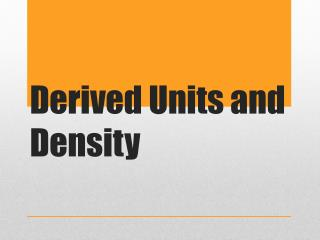Download PresentationDerived Units and Density

# Derived Units and Density

Download Presentation## Derived Units and Density

- - - - - - - - - - - - - - - - - - - - - - - - - - - E N D - - - - - - - - - - - - - - - - - - - - - - - - - - -
##### Presentation Transcript

1. Derived Units and Density

2. A piece of metal has the mass of 3.45 kg. What is its mass in g? • A container has 2.3 L of gas in it? What is its volume in mL? • A container has 750.00 mL of liquid in it. What is its volume in dL? Bell Ringer…

3. Many SI units are combinations of quantities • These units are produced by multiplying or dividing standard units Derived units…

4. Derived SI units…

5. The measure of the resistance of an object to a change in its state of motion OR the amount of “stuff” in an object • A scale is used to mass an object Mass

6. An important point concerning measurements is the relationship between mass and weight • Weight is the force gravity exerts on mass, therefore weight varies with the strength of the gravitational field • Therefore if you went to the moon your weight would change but not your mass • Many times the terms mass and weight are sometimes used interchangeably, although this is incorrect! Mass vs. weight…

7. The derived SI unit of volume is cubic meters (m3) • Many times this unit is way too large to be a practical way of expressing volume in a chemistry lab • Instead, a smaller unit cubic centimeters (cm3) is used • When dealing with the volumes of liquids and gases, the non-SI unit liter(L) is often used • Again the liter is often too large so the unit milliliter (mL) is used • This means 1 cm3 = 1mL Volume…

8. How many mL are in 14.65 kL? • How many L are in 48.6 cm3? • How many dm3 are in 29100 mL? It can be tricky with volume conversions…

9. Ratio of mass to volume • D = m/V • Unit usually: g/mL or g/cm3 • Useful for predicting mass • Does not depend on the amount of material in a compound Density…

10. A characteristic physical property of a substance • It does not depend on the size of the sample because as the sample’s mass increases, its volume increases proportionally • Density varies with temperature – V ↑ T↑ D↓ Density…

11. What is the density of an object that has a mass of 14 g and a volume of 2 ml? • What is the mass of an object with a volume of 2 cm3 and a density of 1.5 g/mL? • What is the volume of an object with the mass of 20 kg and a density of 2.5 g/mL? Try a few…

12. An empty container weighs 121.3 g. Filled with carbon tetrachloride (density 1.53 g/cm3) the container weighs 283.2 g. What is the volume of the container? • A 55.0 gal drum weighs 75 lbs when empty. What will the total mass be when filled with ethanol? density of ethanol = 0.789 g/cm3 1 gal = 3.78 L 1 lb = 454 g Bell Ringer…

13. In the opening scenes of the movie Raiders of the Lost Ark, Indiana Jones tries to remove a gold idol from a booby-trapped pedestal. He replaces the idol with a bag of sand of approximately the same volume. (density of gold = 19.32 g/mL; density or sand = 2 g/mL) • Did he have a reasonable chance of not activating the mass sensitive bobby-trap? • In a later scene he and an unscrupulous guide play catch with the idol. Assume the volume of the idol is 1.0 L. If it were solid gold, what mass would the idol have? • Is playing catch plausible?